What does it do?

Subtracts two numbers

Formula breakdown:

=number1 – number2

What it means:

=the number being deducted from – the number being deducted

In Excel subtracting numbers together is really easy, the Subtraction Formula is actually through the use of the subtraction operator:

I will show you in the steps below how you could use this.

This is the table of values that we want to perform subtraction on: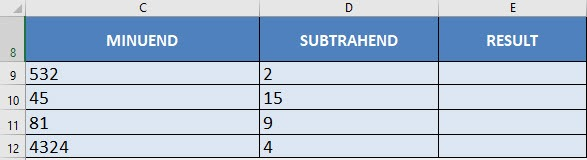I explain how you can do this below:STEP 1: We need to enter the number we want to subtract:

## =C9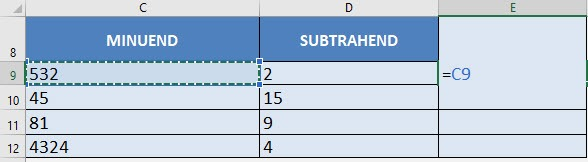STEP 2: Enter the subtraction operator

## =C9 –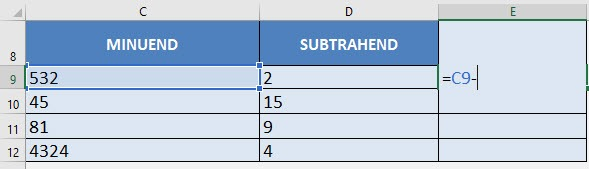STEP 3: Enter the number doing the subtracting:

## =C9 – D9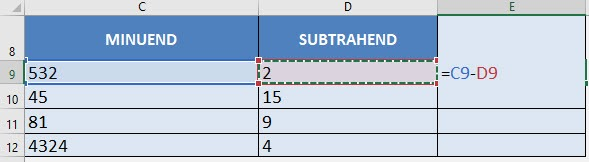Apply the same formula to the rest of the cells by dragging the lower right corner downwards.

You now have all of the subtraction results!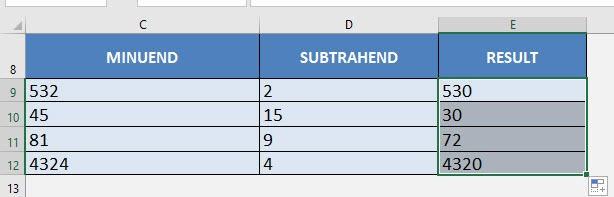How to Use the Subtraction Formula in Excel